Скачать презентацию Electric charges in motion CHAPTER 20 Continuos

bba9e086a493d15d7a0a842550672176.ppt

• Количество слайдов: 12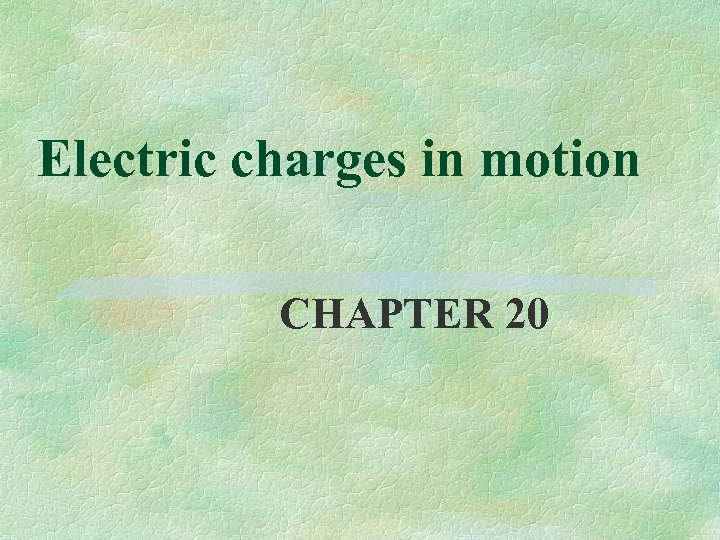Electric charges in motion CHAPTER 20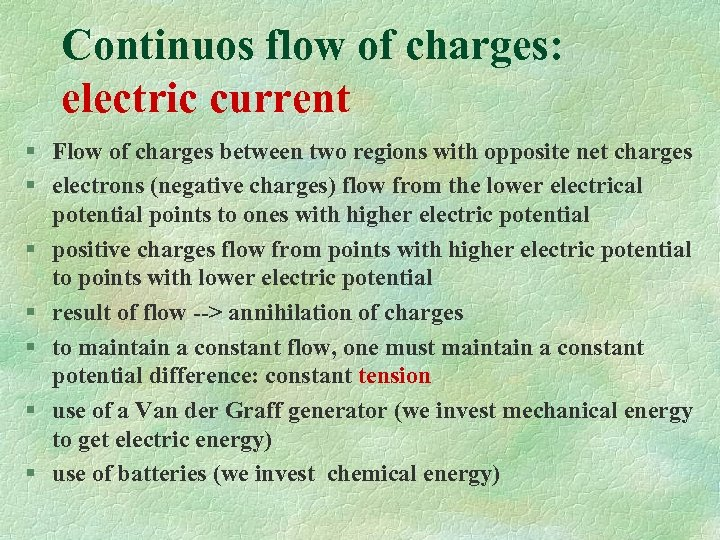Continuos flow of charges: electric current § Flow of charges between two regions with opposite net charges § electrons (negative charges) flow from the lower electrical potential points to ones with higher electric potential § positive charges flow from points with higher electric potential to points with lower electric potential § result of flow --> annihilation of charges § to maintain a constant flow, one must maintain a constant potential difference: constant tension § use of a Van der Graff generator (we invest mechanical energy to get electric energy) § use of batteries (we invest chemical energy)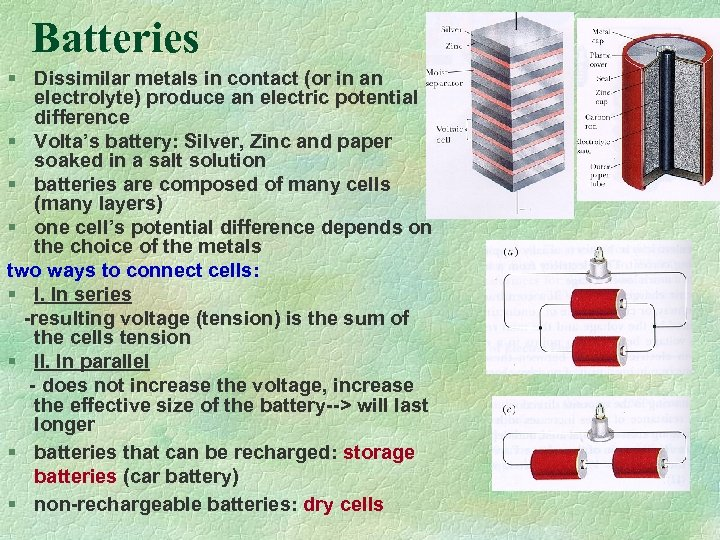Batteries § Dissimilar metals in contact (or in an electrolyte) produce an electric potential difference § Volta’s battery: Silver, Zinc and paper soaked in a salt solution § batteries are composed of many cells (many layers) § one cell’s potential difference depends on the choice of the metals two ways to connect cells: § I. In series -resulting voltage (tension) is the sum of the cells tension § II. In parallel - does not increase the voltage, increase the effective size of the battery--> will last longer § batteries that can be recharged: storage batteries (car battery) § non-rechargeable batteries: dry cells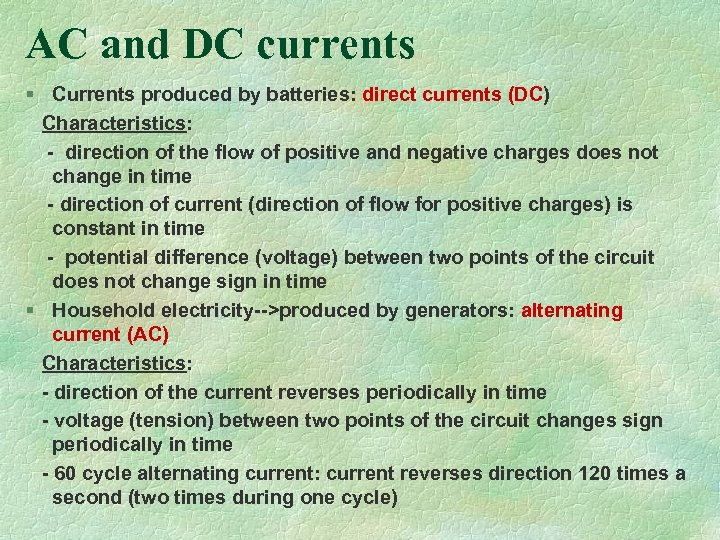AC and DC currents § Currents produced by batteries: direct currents (DC) Characteristics: - direction of the flow of positive and negative charges does not change in time - direction of current (direction of flow for positive charges) is constant in time - potential difference (voltage) between two points of the circuit does not change sign in time § Household electricity-->produced by generators: alternating current (AC) Characteristics: - direction of the current reverses periodically in time - voltage (tension) between two points of the circuit changes sign periodically in time - 60 cycle alternating current: current reverses direction 120 times a second (two times during one cycle)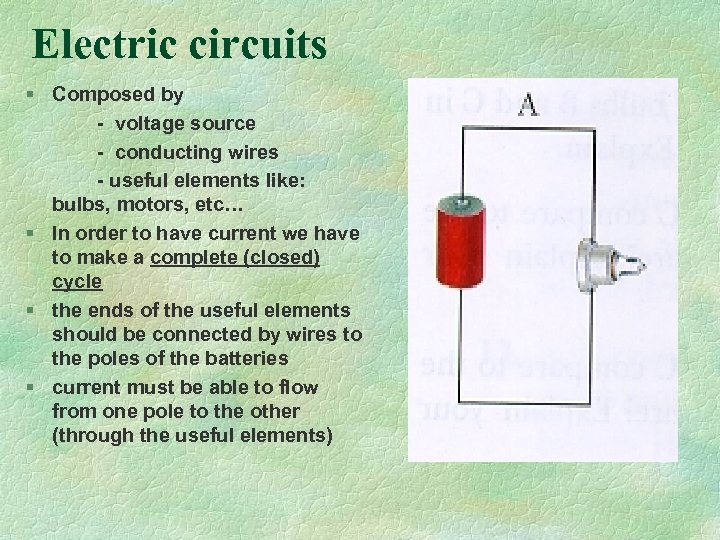Electric circuits § Composed by - voltage source - conducting wires - useful elements like: bulbs, motors, etc… § In order to have current we have to make a complete (closed) cycle § the ends of the useful elements should be connected by wires to the poles of the batteries § current must be able to flow from one pole to the other (through the useful elements)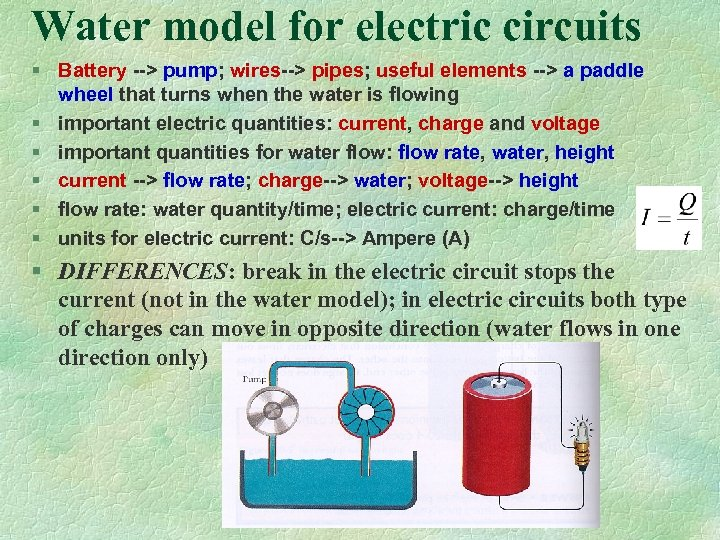Water model for electric circuits § Battery --> pump; wires--> pipes; useful elements --> a paddle wheel that turns when the water is flowing § important electric quantities: current, charge and voltage § important quantities for water flow: flow rate, water, height § current --> flow rate; charge--> water; voltage--> height § flow rate: water quantity/time; electric current: charge/time § units for electric current: C/s--> Ampere (A) § DIFFERENCES: break in the electric circuit stops the current (not in the water model); in electric circuits both type of charges can move in opposite direction (water flows in one direction only)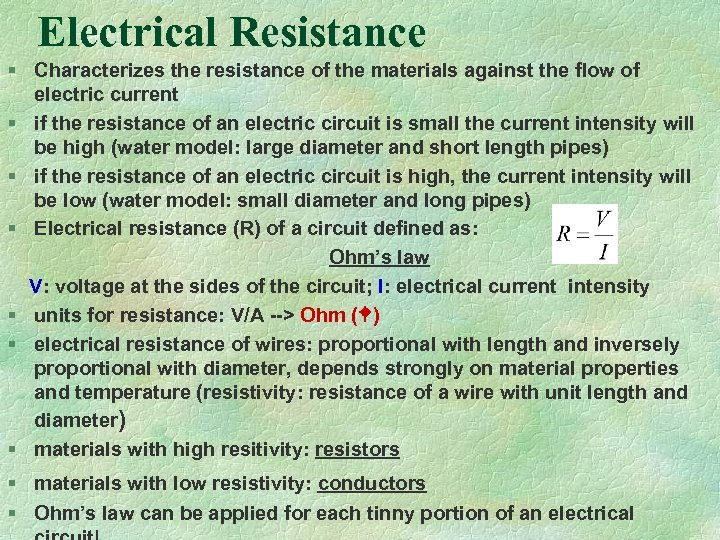Electrical Resistance § Characterizes the resistance of the materials against the flow of electric current § if the resistance of an electric circuit is small the current intensity will be high (water model: large diameter and short length pipes) § if the resistance of an electric circuit is high, the current intensity will be low (water model: small diameter and long pipes) § Electrical resistance (R) of a circuit defined as: Ohm’s law V: voltage at the sides of the circuit; I: electrical current intensity § units for resistance: V/A --> Ohm ( ) § electrical resistance of wires: proportional with length and inversely proportional with diameter, depends strongly on material properties and temperature (resistivity: resistance of a wire with unit length and diameter) § materials with high resitivity: resistors § materials with low resistivity: conductors § Ohm’s law can be applied for each tinny portion of an electrical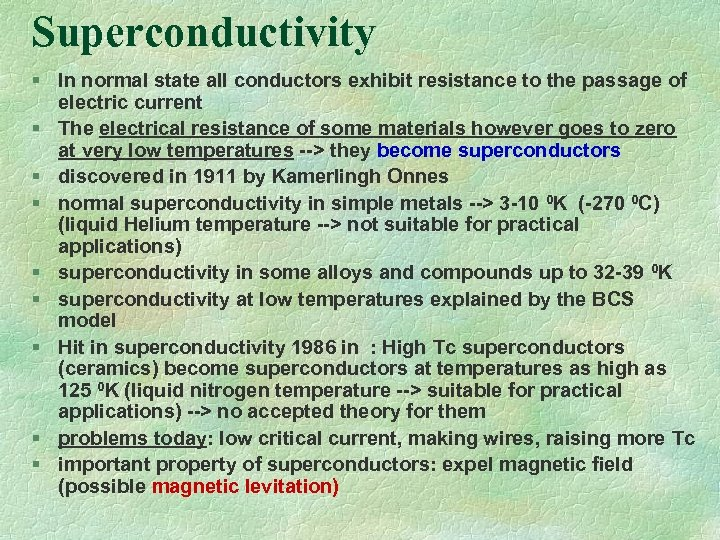Superconductivity § In normal state all conductors exhibit resistance to the passage of electric current § The electrical resistance of some materials however goes to zero at very low temperatures --> they become superconductors § discovered in 1911 by Kamerlingh Onnes § normal superconductivity in simple metals --> 3 -10 0 K (-270 0 C) (liquid Helium temperature --> not suitable for practical applications) § superconductivity in some alloys and compounds up to 32 -39 0 K § superconductivity at low temperatures explained by the BCS model § Hit in superconductivity 1986 in : High Tc superconductors (ceramics) become superconductors at temperatures as high as 125 0 K (liquid nitrogen temperature --> suitable for practical applications) --> no accepted theory for them § problems today: low critical current, making wires, raising more Tc § important property of superconductors: expel magnetic field (possible magnetic levitation)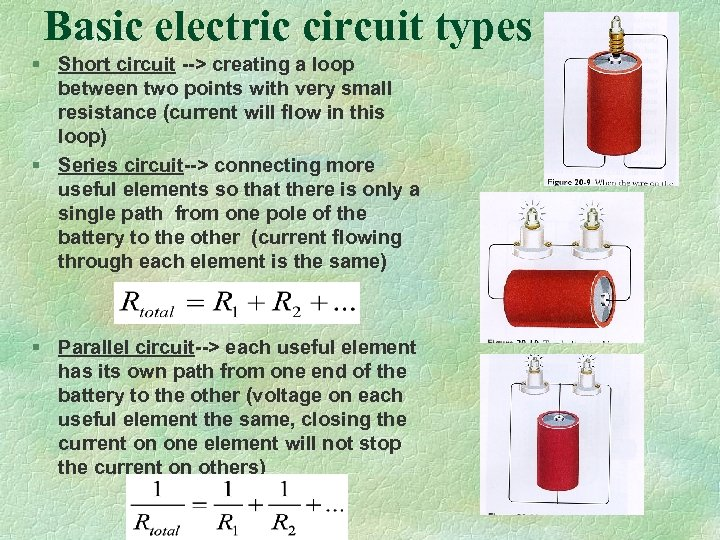Basic electric circuit types § Short circuit --> creating a loop between two points with very small resistance (current will flow in this loop) § Series circuit--> connecting more useful elements so that there is only a single path from one pole of the battery to the other (current flowing through each element is the same) § Parallel circuit--> each useful element has its own path from one end of the battery to the other (voltage on each useful element the same, closing the current on one element will not stop the current on others)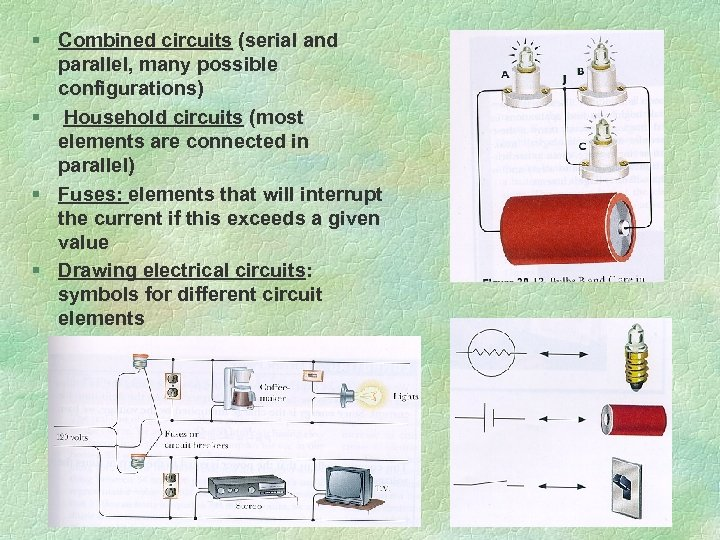§ Combined circuits (serial and parallel, many possible configurations) § Household circuits (most elements are connected in parallel) § Fuses: elements that will interrupt the current if this exceeds a given value § Drawing electrical circuits: symbols for different circuit elements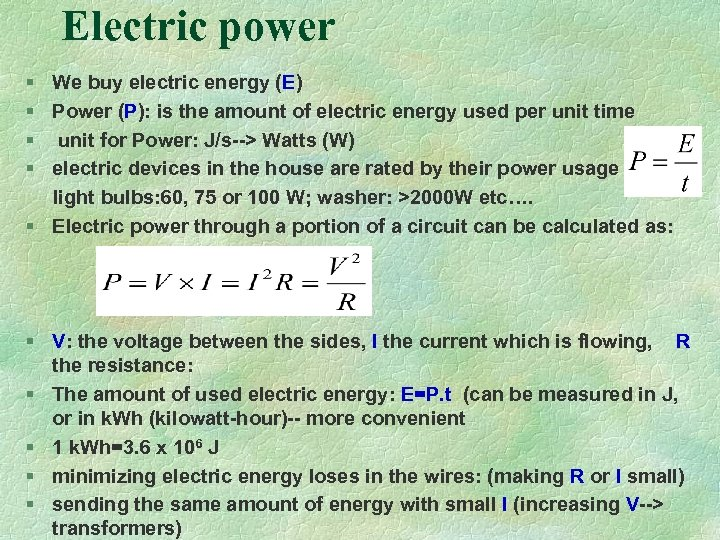Electric power § We buy electric energy (E) § Power (P): is the amount of electric energy used per unit time § unit for Power: J/s--> Watts (W) § electric devices in the house are rated by their power usage light bulbs: 60, 75 or 100 W; washer: >2000 W etc…. § Electric power through a portion of a circuit can be calculated as: § V: the voltage between the sides, I the current which is flowing, R the resistance: § The amount of used electric energy: E=P. t (can be measured in J, or in k. Wh (kilowatt-hour)-- more convenient § 1 k. Wh=3. 6 x 106 J § minimizing electric energy loses in the wires: (making R or I small) § sending the same amount of energy with small I (increasing V--> transformers)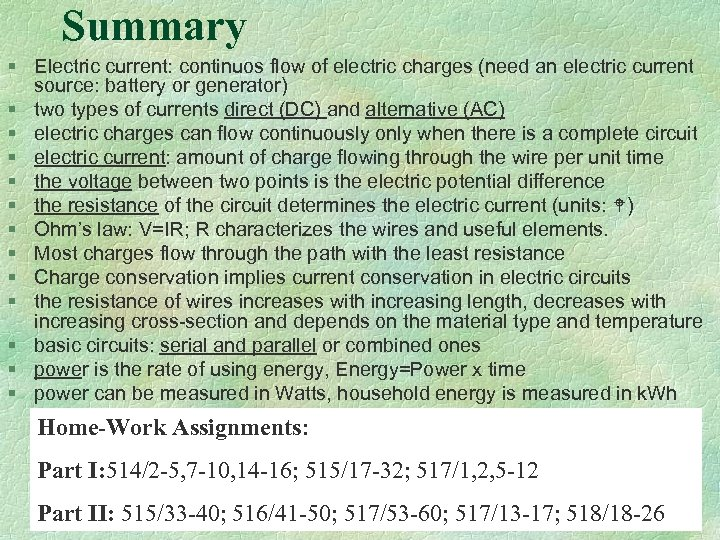Summary § Electric current: continuos flow of electric charges (need an electric current source: battery or generator) § two types of currents direct (DC) and alternative (AC) § electric charges can flow continuously only when there is a complete circuit § electric current: amount of charge flowing through the wire per unit time § the voltage between two points is the electric potential difference § the resistance of the circuit determines the electric current (units: ) § Ohm’s law: V=IR; R characterizes the wires and useful elements. § Most charges flow through the path with the least resistance § Charge conservation implies current conservation in electric circuits § the resistance of wires increases with increasing length, decreases with increasing cross-section and depends on the material type and temperature § basic circuits: serial and parallel or combined ones § power is the rate of using energy, Energy=Power x time § power can be measured in Watts, household energy is measured in k. Wh Home-Work Assignments: Part I: 514/2 -5, 7 -10, 14 -16; 515/17 -32; 517/1, 2, 5 -12 Part II: 515/33 -40; 516/41 -50; 517/53 -60; 517/13 -17; 518/18 -26